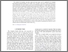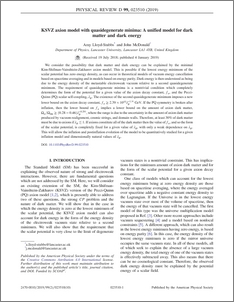# KSVZ axion model with quasidegenerate minima:A unified model for dark matter and dark energy

Lloyd-Stubbs, Amy and McDonald, John (2019) KSVZ axion model with quasidegenerate minima:A unified model for dark matter and dark energy. Physical Review D, 99 (2). ISSN 1550-7998Preview
PDF (PhysRevD.99.023510)
PhysRevD.99.023510.pdf - Published Version

## Abstract

We consider the possibility that dark matter and dark energy can be explained by the minimal Kim-Shifman-Vainshtein-Zakharov axion model. This is possible if the lowest energy minimum of the scalar potential has zero energy density, as can occur in theoretical models of vacuum energy cancellation based on spacetime averaging and in models based on energy parity. Dark energy is then understood as being due to the energy density of the metastable electroweak vacuum relative to a second quasidegenerate minimum. The requirement of quasidegenerate minima is a nontrivial condition which completely determines the form of the potential for a given value of the axion decay constant, fa, and the Pecci-Quinn (PQ) scalar self-coupling, λϕ. The existence of the second quasidegenerate minimum imposes a new lower bound on the axion decay constant, fa ≥ 2.39 × 10^{10} λ_{\phi}^{−1/4} GeV. If the PQ symmetry is broken after inflation, then the lower bound on fa implies a lower bound on the amount of axion dark matter, Ω_{a}/Ω_{dm} ≥ (0.28 − 0.46)λ^{−0.291} , where the range is due to the uncertainty in the amount of axion dark matter produced by vacuum realignment, cosmic strings, and domain walls. Therefore, at least 30% of dark matter must be due to axions if λ_{\phi} ≲ 1. If axions constitute all of the dark matter then the value of fa, and so the form of the scalar potential, is completely fixed for a given value of λϕ, with only a weak dependence on λ_{\phi}. This will allow the inflation and postinflation evolution of the model to be quantitatively studied for a given inflation model and dimensionally natural values of λ_{\phi}.

Item Type:
Journal Article
Journal or Publication Title:
Physical Review D
Uncontrolled Keywords:
/dk/atira/pure/subjectarea/asjc/3100
Subjects:
?? COSMOLOGYPHYSICS AND ASTRONOMY(ALL) ??
Departments:
ID Code:
130449
Deposited By:
Deposited On:
18 Jan 2019 15:40
Refereed?:
Yes
Published?:
Published